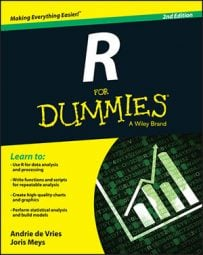##### R For DummiesIn some cases, you don’t have real values to calculate with. In most real-life data sets in R, in fact, at least a few values are missing. Also, some calculations have infinity as a result (such as dividing by zero) or can’t be carried out at all (such as taking the logarithm of a negative value). Luckily, R can deal with all these situations.

## Using infinity

To start exploring infinity in R, see what happens when you try to divide by zero:

```> 2 / 0
 Inf```

R correctly tells you the result is Inf, or infinity. Negative infinity is shown as -Inf. You can use Inf just as you use a real number in calculations:

```> 4 - Inf
 -Inf```

To check whether a value is finite, use the functions is.finite() and is.infinite(). The first function returns TRUE if the number is finite; the second one returns TRUE if the number is infinite.

R considers everything larger than the largest number a computer can hold to be infinity — on most machines, that’s approximately 1.8 × 10308. This definition of infinity can lead to unexpected results, as shown in the following example:

```> is.finite(10^(305:310))
 TRUE TRUE TRUE TRUE FALSE FALSE```

What does this line of code mean now? See whether you understand the nesting and vectorization in this example. If you break up the line starting from the inner parentheses, it becomes comprehensible:

• You know already that 305:310 gives you a vector, containing the integers from 305 to 310.

• All operators are vectorized, so 10^(305:310) gives you a vector with the results of 10 to the power of 305, 306, 307, 308, 309, and 310.

• That vector is given as an argument to is.finite(). This function tells you that the two last results — 10^309 and 10^310 — are infinite for R.

## Dealing with undefined outcomes

Your math teacher probably explained that if you divide any real number by infinity, you get zero. But what if you divide infinity by infinity?

```> Inf / Inf
 NaN```

Well, R tells you that the outcome is NaN. That result simply means Not a Number. This is R’s way of telling you that the outcome of that calculation is not defined.

The funny thing is that R actually considers NaN to be numeric, so you can use NaN in calculations. The outcome of those calculations is always NaN, though, as you see here:

```> NaN + 4
 NaN```

You can test whether a calculation results in NaN by using the is.nan() function. Note that both is.finite() and is.infinite() return FALSE when you’re testing on a NaN value.

## Dealing with missing values

One of the most common problems in statistics is incomplete data sets. To deal with missing values, R uses the reserved keyword NA, which stands for Not Available. You can use NA as a valid value, so you can assign it as a value as well:

`> x <- NA`

You have to take into account, however, that calculations with a value of NA also generally return NA as a result:

```> x + 4
 NA
> log(x)
 NA```

If you want to test whether a value is NA, you can use the is.na() function, as follows:

```> is.na(x)
 TRUE```

Note that the is.na() function also returns TRUE if the value is NaN. The functions is.finite(), is.infinite(), and is.nan() return FALSE for NA values.

## Calculating infinite, undefined, and missing values

The following table provides an overview of results from the functions described above. You are unlikely to use any of these except for is.na(), which you may use quite a lot!

Function Inf –Inf NaN NA
is.finite() FALSE FALSE FALSE FALSE
is.infinite() TRUE TRUE FALSE FALSE
is.nan() FALSE FALSE TRUE FALSE
is.na() FALSE FALSE TRUE TRUE